# Pandas Dataframe: Plot Examples with Matplotlib and Pyplot

Last updated:

All examples can be viewed in this sample Jupyter notebook

You need to have the matplotlib module installed for this!

Versions used: Pandas 1.0.x, matplotlib 3.0.x

### Sample data for examples

import pandas as pd

df = pd.DataFrame({
'name':['john','mary','peter','jeff','bill','lisa','jose'],
'age':[23,78,22,19,45,33,20],
'gender':['M','F','M','M','M','F','M'],
'state':['california','dc','california','dc','california','texas','texas'],
'num_children':[2,0,0,3,2,1,4],
'num_pets':[5,1,0,5,2,2,3]
})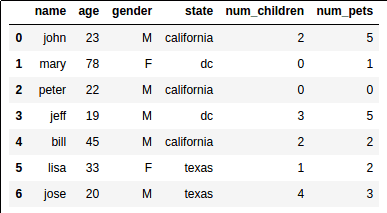This is what our sample dataset looks like

Pandas has tight integration with matplotlib.

You can plot data directly from your DataFrame using the plot() method:

## Scatter plot of two columns

import matplotlib.pyplot as plt
import pandas as pd

# a scatter plot comparing num_children and num_pets
df.plot(kind='scatter',x='num_children',y='num_pets',color='red')
plt.show()Source dataframe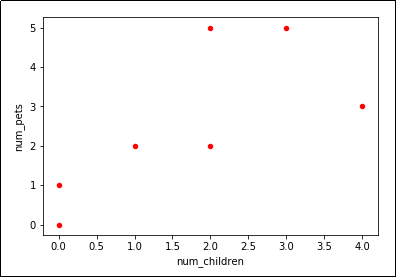Looks like we have a trend

## Bar plot of column values

import matplotlib.pyplot as plt
import pandas as pd

# a simple line plot
df.plot(kind='bar',x='name',y='age')Source dataframe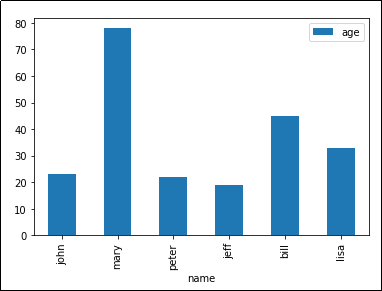'kind' takes arguments such as 'bar', 'barh' (horizontal bars), etc

## Line plot, multiple columns

Just reuse the Axes object.

import matplotlib.pyplot as plt
import pandas as pd

# gca stands for 'get current axis'
ax = plt.gca()

df.plot(kind='line',x='name',y='num_children',ax=ax)
df.plot(kind='line',x='name',y='num_pets', color='red', ax=ax)

plt.show()Source dataframe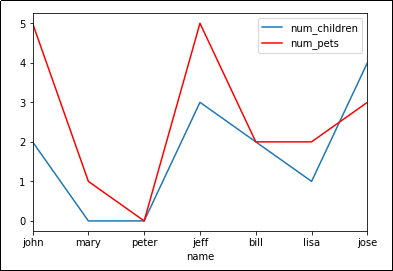plot() takes an optional argument 'ax' which allows you to
reuse an Axis to plot multiple lines

## Save plot to file

Instead of calling plt.show(), call plt.savefig('outputfile.png'):

import matplotlib.pyplot as plt
import pandas as pd

df.plot(kind='bar',x='name',y='age')

# the plot gets saved to 'output.png'
plt.savefig('output.png')


## Bar plot with group by

import matplotlib.pyplot as plt
import pandas as pd

df.groupby('state')['name'].nunique().plot(kind='bar')
plt.show()Source dataframe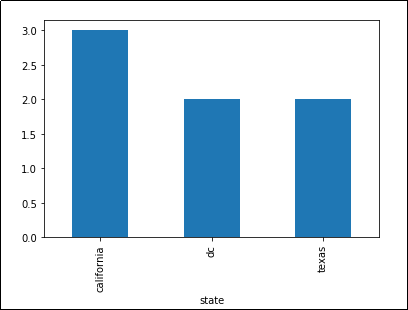Number of unique names per state

## Stacked bar plot with group by

Example: plot count by category as a stacked column:

• create a dummy variable and do a two-level group-by based on it:

• fix the x axis label and the legend

import matplotlib.pyplot as plt

# create dummy variable them group by that
# set the legend to false because we'll fix it later
df.assign(dummy = 1).groupby(
['dummy','state']
).size().to_frame().unstack().plot(kind='bar',stacked=True,legend=False)

plt.title('Number of records by State')

# other it'll show up as 'dummy'
plt.xlabel('state')

# disable ticks in the x axis
plt.xticks([])

# fix the legend
current_handles, _ = plt.gca().get_legend_handles_labels()
reversed_handles = reversed(current_handles)

labels = reversed(df['state'].unique())

plt.legend(reversed_handles,labels,loc='lower right')
plt.show()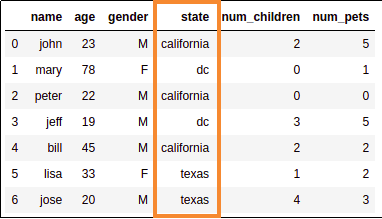3 rows for California,
2 for DC and texas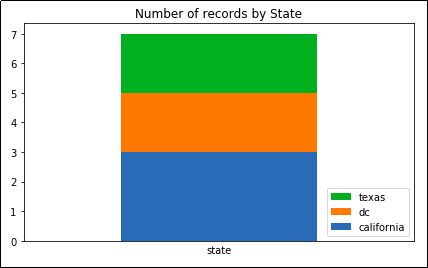Note how the legend follows the
same order as the actual column.

## Stacked bar plot with group by, normalized to 100%

A plot where the columns sum up to 100%.

Similar to the example above but:

• normalize the values by dividing by the total amounts

• use percentage tick labels for the y axis

Example: Plot percentage count of records by state

import matplotlib.pyplot as plt
import matplotlib.ticker as mtick

# create dummy variable then group by that
# set the legend to false because we'll fix it later
df.assign(dummy = 1).groupby(
['dummy','state']
).size().groupby(level=0).apply(
lambda x: 100 * x / x.sum()
).to_frame().unstack().plot(kind='bar',stacked=True,legend=False)

# or it'll show up as 'dummy'
plt.xlabel('state')

# disable ticks in the x axis
plt.xticks([])

# fix the legend or it'll include the dummy variable
current_handles, _ = plt.gca().get_legend_handles_labels()
reversed_handles = reversed(current_handles)
correct_labels = reversed(df['state'].unique())

plt.legend(reversed_handles,correct_labels)

plt.gca().yaxis.set_major_formatter(mtick.PercentFormatter())
plt.show()Source dataframe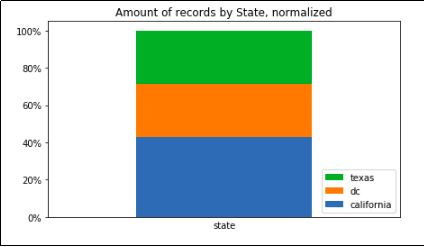Record count grouped by state only, summing up to 100%

## Stacked bar plot, two-level group by

Just do a normal groupby() and call unstack():

import matplotlib.pyplot as plt
import pandas as pd

df.groupby(['state','gender']).size().unstack().plot(kind='bar',stacked=True)
plt.show()Source dataframeStacked bar chart showing the number of people
per state, split into males and females

Another example: count the people by gender, spliting by state:

import matplotlib.pyplot as plt
import pandas as pd

df.groupby(['gender','state']).size().unstack().plot(kind='bar',stacked=True)
plt.show()Source dataframe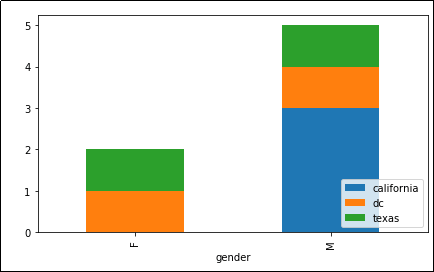Now grouped by 'state' and 'gender'

## Stacked bar plot with two-level group by, normalized to 100%

Sometimes you are only ever interested in the distributions, not raw amounts:

import matplotlib.ticker as mtick
import matplotlib.pyplot as plt

df.groupby(['gender','state']).size().groupby(level=0).apply(
lambda x: 100 * x / x.sum()
).unstack().plot(kind='bar',stacked=True)

plt.gca().yaxis.set_major_formatter(mtick.PercentFormatter())
plt.show()Source dataframe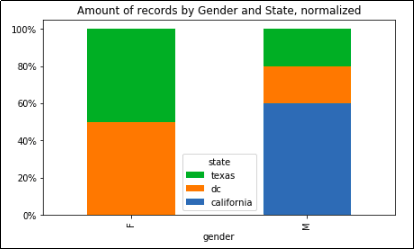Record count grouped by state and gender, with normalized columns
so that each sums up to 100%

## Histogram of column values

You can also use numpy arange to create bins automatically: np.arange(<start>,<stop>,<step>)

import matplotlib.pyplot as plt
import pandas as pd

df[['age']].plot(kind='hist',bins=[0,20,40,60,80,100],rwidth=0.8)
plt.show()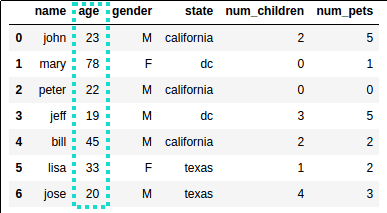Source dataframe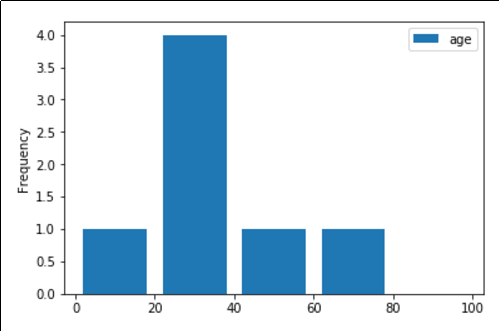The most common age group is between 20 and 40 years old

## Date histogram

To convert from custom formats see: Column to datetime: custom format

To plot the number of records per unit of time, you must a) convert the date column to datetime using to_datetime() b) call .plot(kind='hist'):

import pandas as pd
import matplotlib.pyplot as plt

# source dataframe using an arbitrary date format (m/d/y)
df = pd.DataFrame({
'name':[
'john','lisa','peter','carl','linda','betty'
],
'date_of_birth':[
'01/21/1988','03/10/1977','07/25/1999','01/22/1977','09/30/1968','09/15/1970'
]
})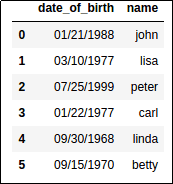Original dataframe, using strings for dates (in American format)

Now convert the date column into datetime type and use plot(kind='hist'):

df['date_of_birth'] = pd.to_datetime(df['date_of_birth'], infer_datetime_format=True)

plt.clf()
df['date_of_birth'].map(lambda d: d.month).plot(kind='hist')
plt.show()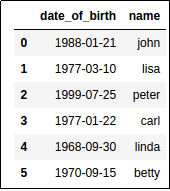The column is now of type datetime64[ns]
(Even though they still look like strings)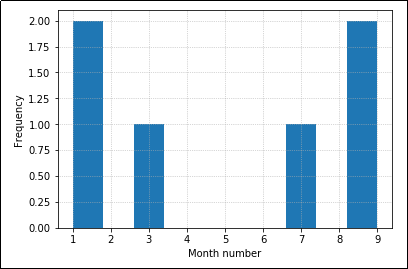Each object is a regular Python datetime.Timestamp object.
Map each one to its month and plot